Publicité

# Digital Logic circuit

1 Feb 2018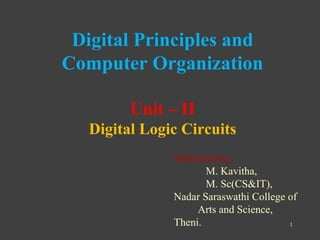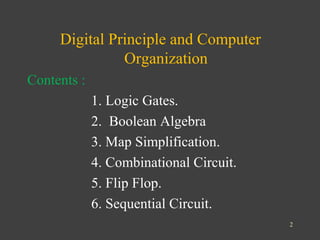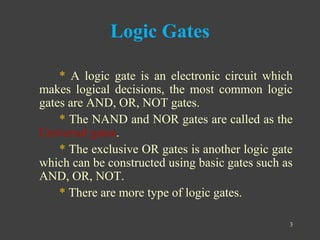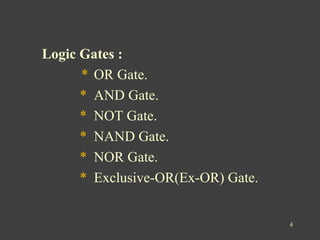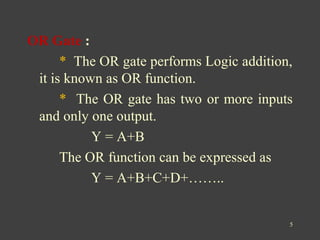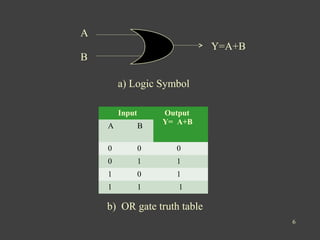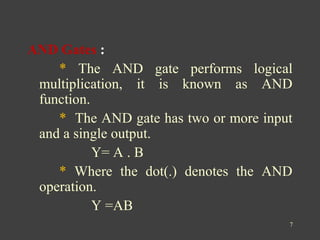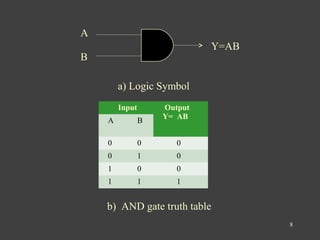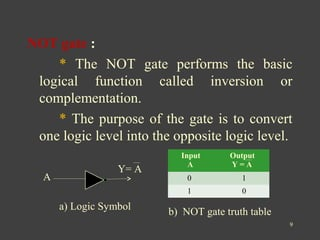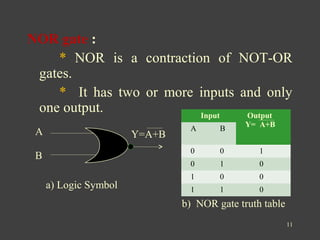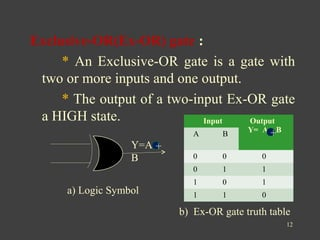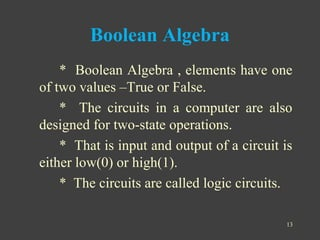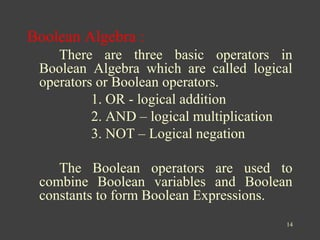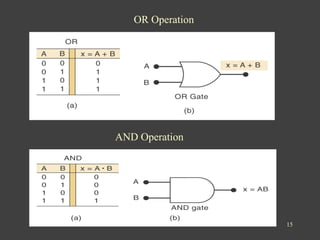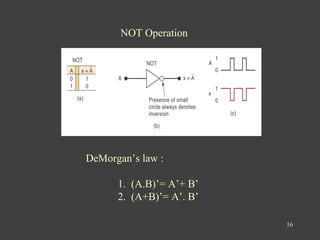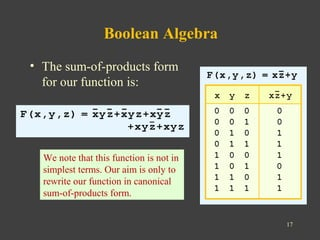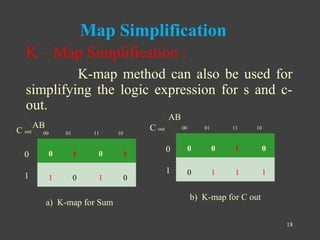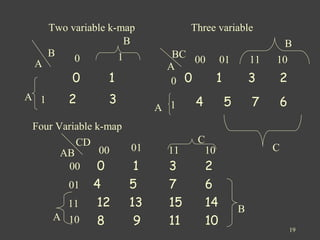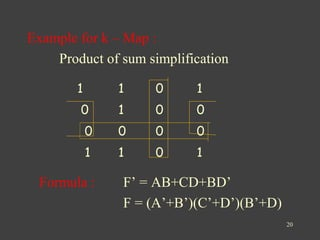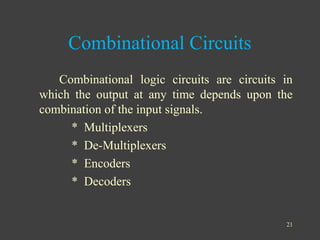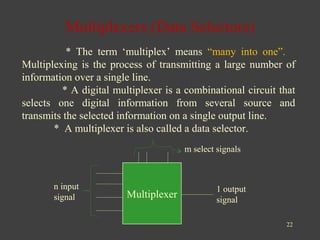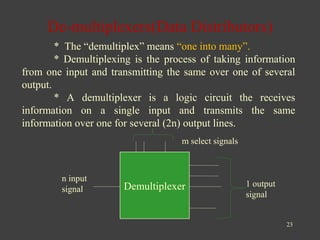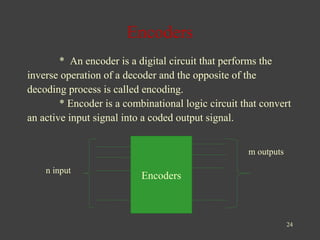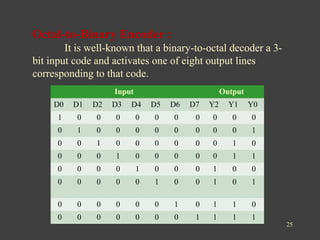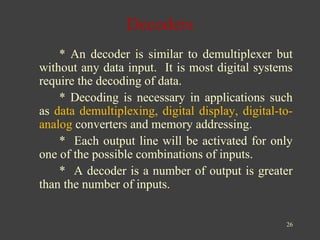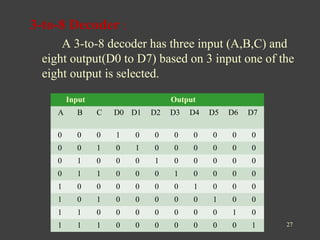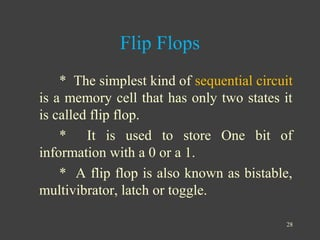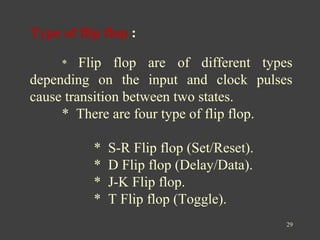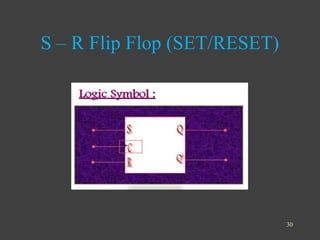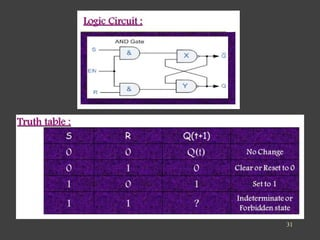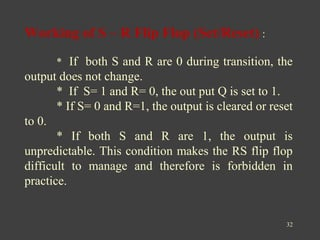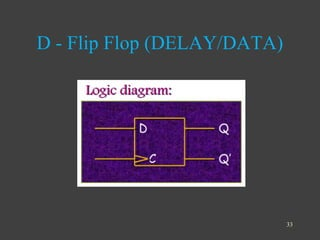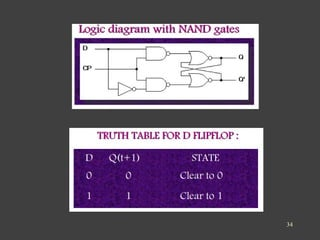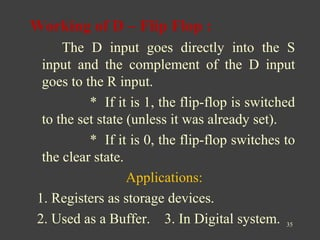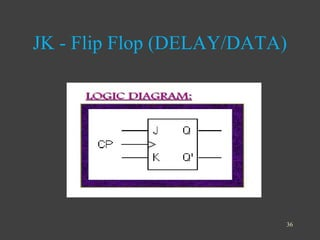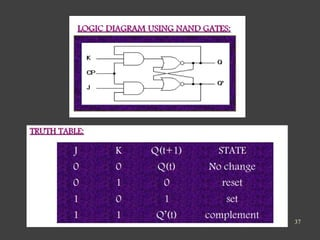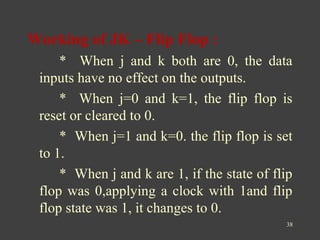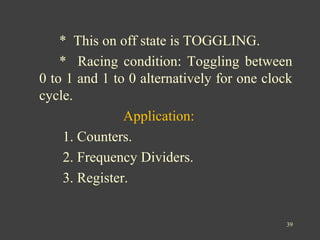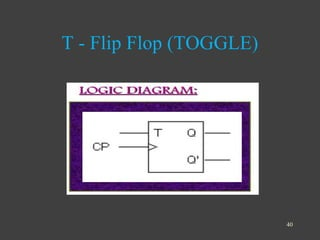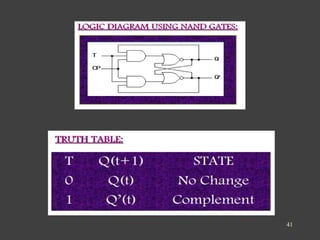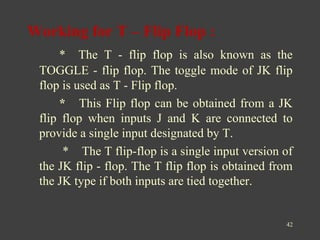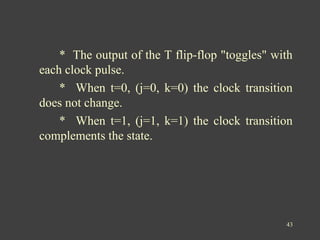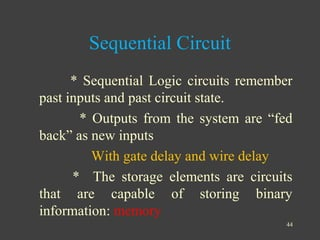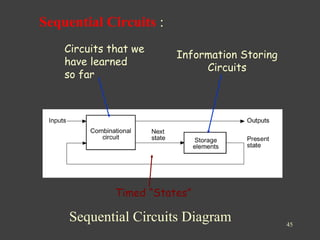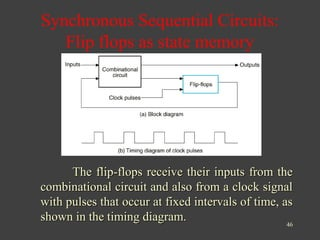1 sur 47
Publicité

### Digital Logic circuit

1. Digital Principles and Computer Organization Unit – II Digital Logic Circuits Submitted by, M. Kavitha, M. Sc(CS&IT), Nadar Saraswathi College of Arts and Science, Theni. 1
2. Digital Principle and Computer Organization Contents : 1. Logic Gates. 2. Boolean Algebra 3. Map Simplification. 4. Combinational Circuit. 5. Flip Flop. 6. Sequential Circuit. 2
3. 3 Logic Gates * A logic gate is an electronic circuit which makes logical decisions, the most common logic gates are AND, OR, NOT gates. * The NAND and NOR gates are called as the Universal gates. * The exclusive OR gates is another logic gate which can be constructed using basic gates such as AND, OR, NOT. * There are more type of logic gates.
4. 4 Logic Gates : * OR Gate. * AND Gate. * NOT Gate. * NAND Gate. * NOR Gate. * Exclusive-OR(Ex-OR) Gate.
5. 5 OR Gate : * The OR gate performs Logic addition, it is known as OR function. * The OR gate has two or more inputs and only one output. Y = A+B The OR function can be expressed as Y = A+B+C+D+……..
6. 6 A B Y=A+B Input Output Y= A+B A B 0 0 0 0 1 1 1 0 1 1 1 1 a) Logic Symbol b) OR gate truth table
7. 7 AND Gates : * The AND gate performs logical multiplication, it is known as AND function. * The AND gate has two or more input and a single output. Y= A . B * Where the dot(.) denotes the AND operation. Y =AB
8. 8 A B Y=AB Input Output Y= AB A B 0 0 0 0 1 0 1 0 0 1 1 1 a) Logic Symbol b) AND gate truth table
9. 9 NOT gate : * The NOT gate performs the basic logical function called inversion or complementation. * The purpose of the gate is to convert one logic level into the opposite logic level. Input A Output Y = A 0 1 1 0 Y= A A a) Logic Symbol b) NOT gate truth table
10. 10 NAND gate : * NAND is a contraction of the NOT-AND gates. * It has two or more inputs and only one output. 10 Input Output Y= AB A B 0 0 1 0 1 1 1 0 1 1 1 0a) Logic Symbol b) NAND gate truth table A B Y=AB
11. 11 NOR gate : * NOR is a contraction of NOT-OR gates. * It has two or more inputs and only one output. A B Y=A+B Input Output Y= A+B A B 0 0 1 0 1 0 1 0 0 1 1 0a) Logic Symbol b) NOR gate truth table
12. 12 Exclusive-OR(Ex-OR) gate : * An Exclusive-OR gate is a gate with two or more inputs and one output. * The output of a two-input Ex-OR gate a HIGH state. Y=A B Input Output Y= A B A B 0 0 0 0 1 1 1 0 1 1 1 0a) Logic Symbol b) Ex-OR gate truth table + +
13. Boolean Algebra * Boolean Algebra , elements have one of two values –True or False. * The circuits in a computer are also designed for two-state operations. * That is input and output of a circuit is either low(0) or high(1). * The circuits are called logic circuits. 13
14. Boolean Algebra : There are three basic operators in Boolean Algebra which are called logical operators or Boolean operators. 1. OR - logical addition 2. AND – logical multiplication 3. NOT – Logical negation The Boolean operators are used to combine Boolean variables and Boolean constants to form Boolean Expressions. 14
15. 15 OR Operation AND Operation
16. 16 NOT Operation DeMorgan’s law : 1. (A.B)’= A’+ B’ 2. (A+B)’= A’. B’
17. 17 Boolean Algebra • The sum-of-products form for our function is: We note that this function is not in simplest terms. Our aim is only to rewrite our function in canonical sum-of-products form.
18. 18 Map Simplification K – Map Simplification : K-map method can also be used for simplifying the logic expression for s and c- out. 0 1 0 1 1 0 1 0 0 0 1 0 0 1 1 1 AB AB C out C out 00 01 11 10 00 01 11 10 0 1 0 1 a) K-map for Sum b) K-map for C out
19. 19 0 1 2 3 A B 0 B 1A 1 Two variable k-map Three variable 0 1 3 2 4 5 7 6 A BC 00 01 11 10 0 1 B A C Four Variable k-map 0 1 3 2 4 5 7 6 12 13 15 14 8 9 11 10 AB CD 00 01 11 10 00 01 11 10 A B C
20. Example for k – Map : Product of sum simplification Formula : F’ = AB+CD+BD’ F = (A’+B’)(C’+D’)(B’+D) 20 1 1 0 1 0 1 0 0 0 0 0 0 1 1 0 1
21. 21 Combinational Circuits Combinational logic circuits are circuits in which the output at any time depends upon the combination of the input signals. * Multiplexers * De-Multiplexers * Encoders * Decoders
22. 22 Multiplexers (Data Selectors) * The term ‘multiplex’ means “many into one”. Multiplexing is the process of transmitting a large number of information over a single line. * A digital multiplexer is a combinational circuit that selects one digital information from several source and transmits the selected information on a single output line. * A multiplexer is also called a data selector. Multiplexer 1 output signal m select signals n input signal
23. 23 De-multiplexers(Data Distributors) * The “demultiplex” means “one into many”. * Demultiplexing is the process of taking information from one input and transmitting the same over one of several output. * A demultiplexer is a logic circuit the receives information on a single input and transmits the same information over one for several (2n) output lines. Demultiplexer 1 output signal m select signals n input signal
24. 24 Encoders * An encoder is a digital circuit that performs the inverse operation of a decoder and the opposite of the decoding process is called encoding. * Encoder is a combinational logic circuit that convert an active input signal into a coded output signal. Encoders m outputs n input
25. 25 Octal-to-Binary Encoder : It is well-known that a binary-to-octal decoder a 3- bit input code and activates one of eight output lines corresponding to that code. . Input Output D0 D1 D2 D3 D4 D5 D6 D7 Y2 Y1 Y0 1 0 0 0 0 0 0 0 0 0 0 0 1 0 0 0 0 0 0 0 0 1 0 0 1 0 0 0 0 0 0 1 0 0 0 0 1 0 0 0 0 0 1 1 0 0 0 0 1 0 0 0 1 0 0 0 0 0 0 0 1 0 0 1 0 1 0 0 0 0 0 0 1 0 1 1 0 0 0 0 0 0 0 0 1 1 1 1
26. 26 * An decoder is similar to demultiplexer but without any data input. It is most digital systems require the decoding of data. * Decoding is necessary in applications such as data demultiplexing, digital display, digital-to- analog converters and memory addressing. * Each output line will be activated for only one of the possible combinations of inputs. * A decoder is a number of output is greater than the number of inputs. Decoders
27. 3-to-8 Decoder : A 3-to-8 decoder has three input (A,B,C) and eight output(D0 to D7) based on 3 input one of the eight output is selected. 27 Input Output A B C D0 D1 D2 D3 D4 D5 D6 D7 0 0 0 1 0 0 0 0 0 0 0 0 0 1 0 1 0 0 0 0 0 0 0 1 0 0 0 1 0 0 0 0 0 0 1 1 0 0 0 1 0 0 0 0 1 0 0 0 0 0 0 1 0 0 0 1 0 1 0 0 0 0 0 1 0 0 1 1 0 0 0 0 0 0 0 1 0 1 1 1 0 0 0 0 0 0 0 1
28. Flip Flops * The simplest kind of sequential circuit is a memory cell that has only two states it is called flip flop. * It is used to store One bit of information with a 0 or a 1. * A flip flop is also known as bistable, multivibrator, latch or toggle. 28
29. 29 Type of flip flop : * Flip flop are of different types depending on the input and clock pulses cause transition between two states. * There are four type of flip flop. * S-R Flip flop (Set/Reset). * D Flip flop (Delay/Data). * J-K Flip flop. * T Flip flop (Toggle).
30. S – R Flip Flop (SET/RESET) 30
31. 31
32. 32 Working of S – R Flip Flop (Set/Reset) : * If both S and R are 0 during transition, the output does not change. * If S= 1 and R= 0, the out put Q is set to 1. * If S= 0 and R=1, the output is cleared or reset to 0. * If both S and R are 1, the output is unpredictable. This condition makes the RS flip flop difficult to manage and therefore is forbidden in practice.
33. D - Flip Flop (DELAY/DATA) 33
34. 34
35. Working of D – Flip Flop : The D input goes directly into the S input and the complement of the D input goes to the R input. * If it is 1, the flip-flop is switched to the set state (unless it was already set). * If it is 0, the flip-flop switches to the clear state. Applications: 1. Registers as storage devices. 2. Used as a Buffer. 3. In Digital system. 35
36. JK - Flip Flop (DELAY/DATA) 36
37. 37
38. Working of JK – Flip Flop : * When j and k both are 0, the data inputs have no effect on the outputs. * When j=0 and k=1, the flip flop is reset or cleared to 0. * When j=1 and k=0. the flip flop is set to 1. * When j and k are 1, if the state of flip flop was 0,applying a clock with 1and flip flop state was 1, it changes to 0. 38
39. * This on off state is TOGGLING. * Racing condition: Toggling between 0 to 1 and 1 to 0 alternatively for one clock cycle. Application: 1. Counters. 2. Frequency Dividers. 3. Register. 39
40. T - Flip Flop (TOGGLE) 40
41. 41
42. Working for T – Flip Flop : * The T - flip flop is also known as the TOGGLE - flip flop. The toggle mode of JK flip flop is used as T - Flip flop. * This Flip flop can be obtained from a JK flip flop when inputs J and K are connected to provide a single input designated by T. * The T flip-flop is a single input version of the JK flip - flop. The T flip flop is obtained from the JK type if both inputs are tied together. 42
43. * The output of the T flip-flop "toggles" with each clock pulse. * When t=0, (j=0, k=0) the clock transition does not change. * When t=1, (j=1, k=1) the clock transition complements the state. 43
44. Sequential Circuit * Sequential Logic circuits remember past inputs and past circuit state. * Outputs from the system are “fed back” as new inputs With gate delay and wire delay * The storage elements are circuits that are capable of storing binary information: memory 44
45. Sequential Circuits : 45 Sequential Circuits Diagram Circuits that we have learned so far Information Storing Circuits Timed “States”
46. 46 Synchronous Sequential Circuits: Flip flops as state memory The flip-flops receive their inputs from theThe flip-flops receive their inputs from the combinational circuit and also from a clock signalcombinational circuit and also from a clock signal with pulses that occur at fixed intervals of time, aswith pulses that occur at fixed intervals of time, as shown in the timing diagram.shown in the timing diagram.
47. Thank You 47
Publicité